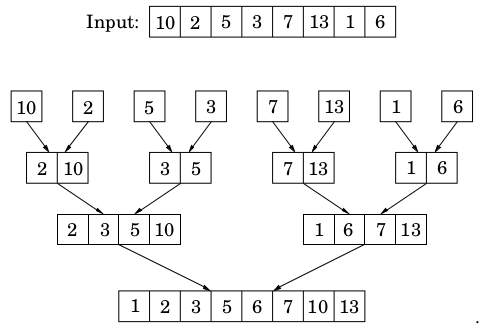# Merge Sort solved by 1194

Feb. 21, 2014, 4:24 p.m. by Rosalind Team

Topics: Divide-and-conquer, Sorting

## Problem

The problem of sorting a list of numbers lends itself immediately to a divide-and-conquer strategy: split the list into two halves, recursively sort each half, and then merge the two sorted sublists (recall the problem “Merge Two Sorted Arrays”).

Given: A positive integer $n \le 10^5$ and an array $A[1..n]$ of integers from $-10^5$ to $10^5$.

Return: A sorted array $A[1..n]$.

## Sample Dataset

10
20 19 35 -18 17 -20 20 1 4 4


## Sample Output

-20 -18 1 4 4 17 19 20 20 35


## Visualization

Visualization by David R. Martin: http://www.sorting-algorithms.com/merge-sort

## Running time

The running time of Merge Sort satisfies a recurrence relation $T(n)=2T(n/2)+O(n)$. The master theorem implies that $T(n)=O(n\log n)$.

## Non-recursive implementation

Looking back at the Merge Sort algorithm, we see that all the real work is done in merging, which doesn’t start until the recursion gets down to singleton arrays. The singletons are merged in pairs, to yield arrays with two elements. Then pairs of these $2$-tuples are merged, producing $4$-tuples, and so on. The following figure shows an example.This viewpoint also suggests how Merge Sort might be made iterative. At any given moment, there is a set of “active” arrays—initially, the singletons—which are merged in pairs to give the next batch of active arrays. These arrays can be organized in a queue, and processed by repeatedly removing two arrays from the front of the queue, merging them, and putting the result at the end of the queue.